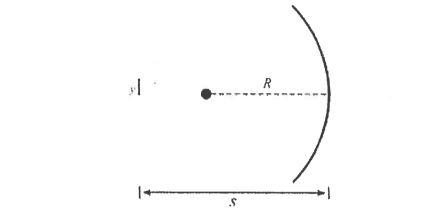# Problem: An object with height y = 2 mm is placed a distance s = 24 cm in front of the vertex of a concave spherical mirror with radius of curvature R = 16 cm, as shown in the figure below. What are the image distance s' and the height y' of the resulting image? s' = _______________ y' = _______________

90% (58 ratings)
###### Problem Details

An object with height y = 2 mm is placed a distance s = 24 cm in front of the vertex of a concave spherical mirror with radius of curvature R = 16 cm, as shown in the figure below. What are the image distance s' and the height y' of the resulting image?

s' = _______________

y' = _______________